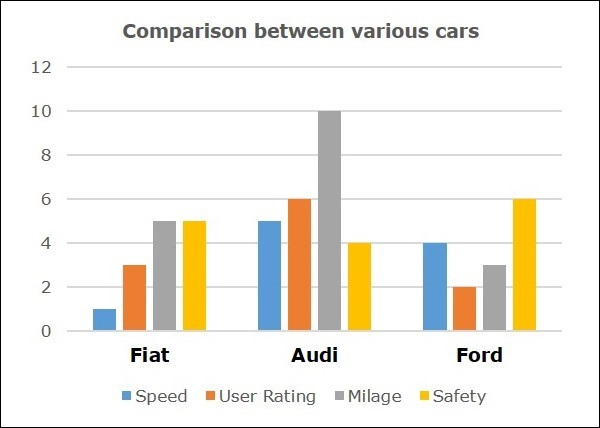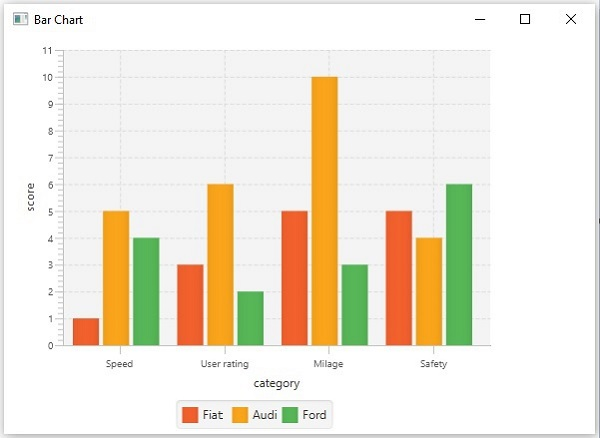# JavaFX - Bar Chart

A bar chart is used to represent grouped data using rectangular bars. The length of these bars depicts the values. The bars in the bar chart can be plotted vertically or horizontally.

Following is a bar chart, comparing various car brands.In JavaFX, a Bar chart is represented by a class named BarChart. This class belongs to the package javafx.scene.chart. By instantiating this class, you can create an BarChart node in JavaFX.

## Steps to Generate Bar Chart

To generate a bar chart in JavaFX, follow the steps given below.

### Step 1: Creating a Class

Create a Java class and inherit the Application class of the package javafx.application. You can then implement the start() method of this class as follows.

```public class ClassName extends Application {
@Override
public void start(Stage primaryStage) throws Exception {
}
}
```

### Step 2: Defining the Axis

Define the X and Y axis of the bar chart and set labels to them. In our example, X axis represents the category of comparison and the y axis represents the score.

```//Defining the x axis
CategoryAxis xAxis = new CategoryAxis();

xAxis.setCategories(FXCollections.<String>observableArrayList(Arrays.asList(
"Speed", "User rating", "Milage", "Safety")));
xAxis.setLabel("category");

//Defining the y axis
NumberAxis yAxis = new NumberAxis();
yAxis.setLabel("score");
```

### Step 3: Creating the Bar Chart

Create a line chart by instantiating the class named BarChart of the package javafx.scene.chart. To the constructor of this class, pass the objects representing the X and Y axis created in the previous step.

```//Creating the Bar chart
BarChart<String, Number> barChart = new BarChart<>(xAxis, yAxis);
barChart.setTitle("Comparison between various cars");
```

### Step 4: Preparing the Data

Instantiate the XYChart.Series class and add the data (a series of, x and y coordinates) to the Observable list of this class as follows −

```//Prepare XYChart.Series objects by setting data
XYChart.Series<String, Number> series1 = new XYChart.Series<>();
series1.setName("Fiat");

XYChart.Series<String, Number> series2 = new XYChart.Series<>();
series2.setName("Audi");

XYChart.Series<String, Number> series3 = new XYChart.Series<>();
series3.setName("Ford");
```

### Step 5: Add Data to the Bar Chart

Add the data series prepared in the previous step to the bar chart as follows −

```//Setting the data to bar chart
```

### Step 6: Creating a Group Object

In the start() method, create a group object by instantiating the class named Group. This belongs to the package javafx.scene.

Pass the BarChart (node) object, created in the previous step as a parameter to the constructor of the Group class. This should be done in order to add it to the group as follows −

```Group root = new Group(barChart);
```

### Step 7: Creating a Scene Object

Create a Scene by instantiating the class named Scene, which belongs to the package javafx.scene. To this class, pass the Group object (root) created in the previous step.

In addition to the root object, you can also pass two double parameters representing height and width of the screen, along with the object of the Group class as follows.

```Scene scene = new Scene(group ,600, 300);
```

### Step 8: Setting the Title of the Stage

You can set the title to the stage using the setTitle() method of the Stage class. The primaryStage is a Stage object, which is passed to the start method of the scene class as a parameter.

Using the primaryStage object, set the title of the scene as Sample Application as follows.

```primaryStage.setTitle("Sample Application");
```

### Step 9: Adding Scene to the Stage

You can add a Scene object to the stage using the method setScene() of the class named Stage. Add the Scene object prepared in the previous steps using the following method.

```primaryStage.setScene(scene);
```

### Step 10: Displaying the Contents of the Stage

Display the contents of the scene using the method named show() of the Stage class as follows.

```primaryStage.show();
```

### Step 11: Launching the Application

Launch the JavaFX application by calling the static method launch() of the Application class from the main method as follows.

```public static void main(String args[]){
launch(args);
}
```

### Example

The following example depicts various car statistics with the help of a bar chart. Following is a list of car brands along with their different characteristics, which we will show using a bar chart −

Car Speed User Rating Millage Safety
Fiat 1.0 3.0 5.0 5.0
Audi 5.0 6.0 10.0 4.0
Ford 4.0 2.0 3.0 6.0

Following is a Java program which generates a bar chart, depicting the above data using JavaFX.

Save this code in a file with the name BarChartExample.java.

```import java.util.Arrays;
import javafx.application.Application;
import javafx.collections.FXCollections;
import javafx.scene.Group;
import javafx.scene.Scene;
import javafx.scene.chart.BarChart;
import javafx.scene.chart.CategoryAxis;
import javafx.stage.Stage;
import javafx.scene.chart.NumberAxis;
import javafx.scene.chart.XYChart;

public class BarChartExample extends Application {
@Override
public void start(Stage stage) {
//Defining the axes
CategoryAxis xAxis = new CategoryAxis();
xAxis.setCategories(FXCollections.<String>
observableArrayList(Arrays.asList("Speed", "User rating", "Milage", "Safety")));
xAxis.setLabel("category");

NumberAxis yAxis = new NumberAxis();
yAxis.setLabel("score");

//Creating the Bar chart
BarChart<String, Number> barChart = new BarChart<>(xAxis, yAxis);
barChart.setTitle("Comparison between various cars");

//Prepare XYChart.Series objects by setting data
XYChart.Series<String, Number> series1 = new XYChart.Series<>();
series1.setName("Fiat");

XYChart.Series<String, Number> series2 = new XYChart.Series<>();
series2.setName("Audi");

XYChart.Series<String, Number> series3 = new XYChart.Series<>();
series3.setName("Ford");

//Setting the data to bar chart

//Creating a Group object
Group root = new Group(barChart);

//Creating a scene object
Scene scene = new Scene(root, 600, 400);

//Setting title to the Stage
stage.setTitle("Bar Chart");

stage.setScene(scene);

//Displaying the contents of the stage
stage.show();
}
public static void main(String args[]){
launch(args);
}
}
```

Compile and execute the saved java file from the command prompt using the following commands.

```javac BarChartExample.java
java BarChartExample
```

On executing, the above program generates a JavaFX window displaying an area chart as shown below.javafx_charts.htm